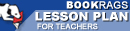Lesson Plans
Related Topics

# Daily Lessons for Teaching Thirteen Reasons Why

This set of Lesson Plans consists of approximately 117 pages of tests, essay questions, lessons, and other teaching materials.
 View a FREE sample## Lesson 1 (from Prologue)

### Objective

Every story begins with an introduction where the tone, characters and plot of the story are introduced. The introduction serves to whet the appetite of the reader and hook them on the story, thus making them want to read further. The objective of this lesson is to examine the introduction and set-up of the novel.

### Lesson

Class Discussion: Imagine a general plot line. Where is the introduction of a story on that line? What is the purpose of an introduction? Do all stories have one? How does the introduction serve the reader? What happens when a story has a poor, or uninteresting, introduction?

Individual Assignment: Draw a plot line. Make sure sections are labeled for: Introduction, Rising Action, Climax, Falling Action and Resolution. Under the Introduction segment, write in 2 -3 specific incidents from the Prologue that you found to be compelling. Save this graph for use...

 This section contains 8,200 words (approx. 28 pages at 300 words per page) View a FREE sample